# 如何實現一個高效的Softmax CUDA kernel？

Softmax操作是深度學習模型中最常用的操作之一。在深度學習的分類任務中，網路最後的分類器往往是Softmax + CrossEntropy的組合：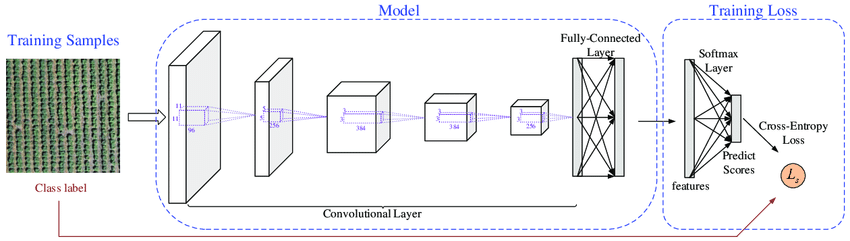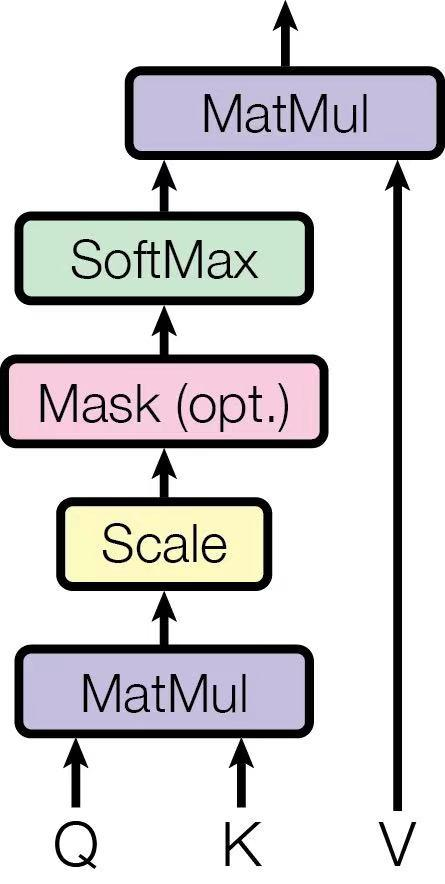\

# GPU基礎知識與CUDA效能優化原則

\

https://zhuanlan.zhihu.com/p/271740706

\

• GPU記憶體分為Global memory、Shared memory、Local memory三級。
• GPU最主要提供的是兩種資源：計算資源 和 視訊記憶體頻寬資源。如果我們能將這兩種資源充分利用，且對資源的需求無法再降低，那麼效能就優化到了極限，執行時間就會最短。在大多數情況下，深度學習訓練中的GPU計算資源是被充分利用的，而一個GPU CUDA Kernel的優化目標就是儘可能充分利用視訊記憶體頻寬資源。

# Naive的Softmax實現：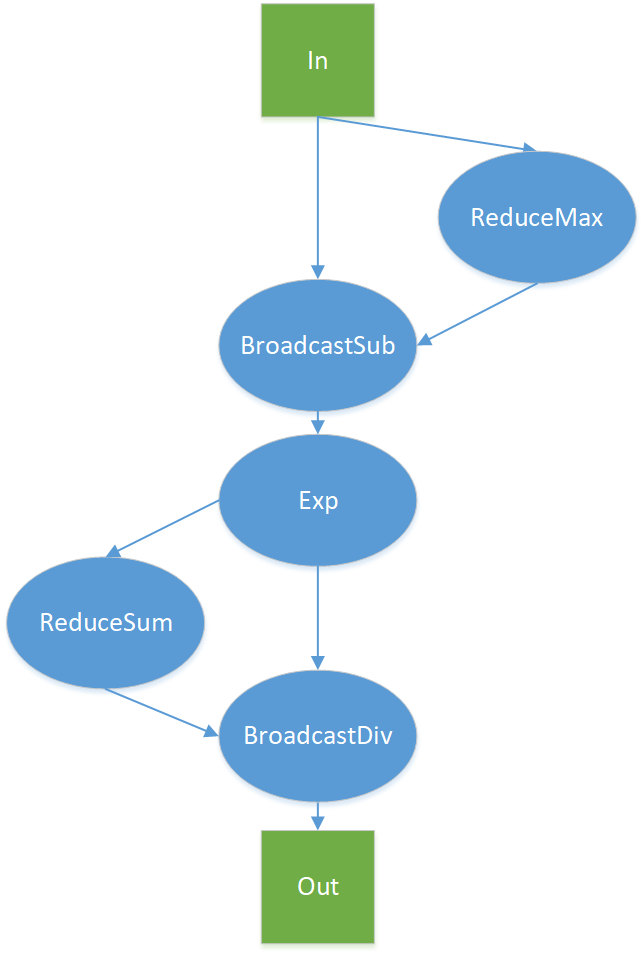• ReduceMax = D + num_rows （read 為 D， write 為 num_rows）
• BroadcaseSub = 2 * D + num_rows （read 為 D + num_rows，write 為 D）
• Exp = 2 * D （read 、write 均為D）
• ReduceSum = D + num_rows（read 為 D， write 為 num_rows）
• BroadcastDive = 2 * D + num_rows （read 為 D + num_rows， write 為 D）

# OneFlow 與 cuDNN 的對比結果

Softmax Kernel Bandwidth：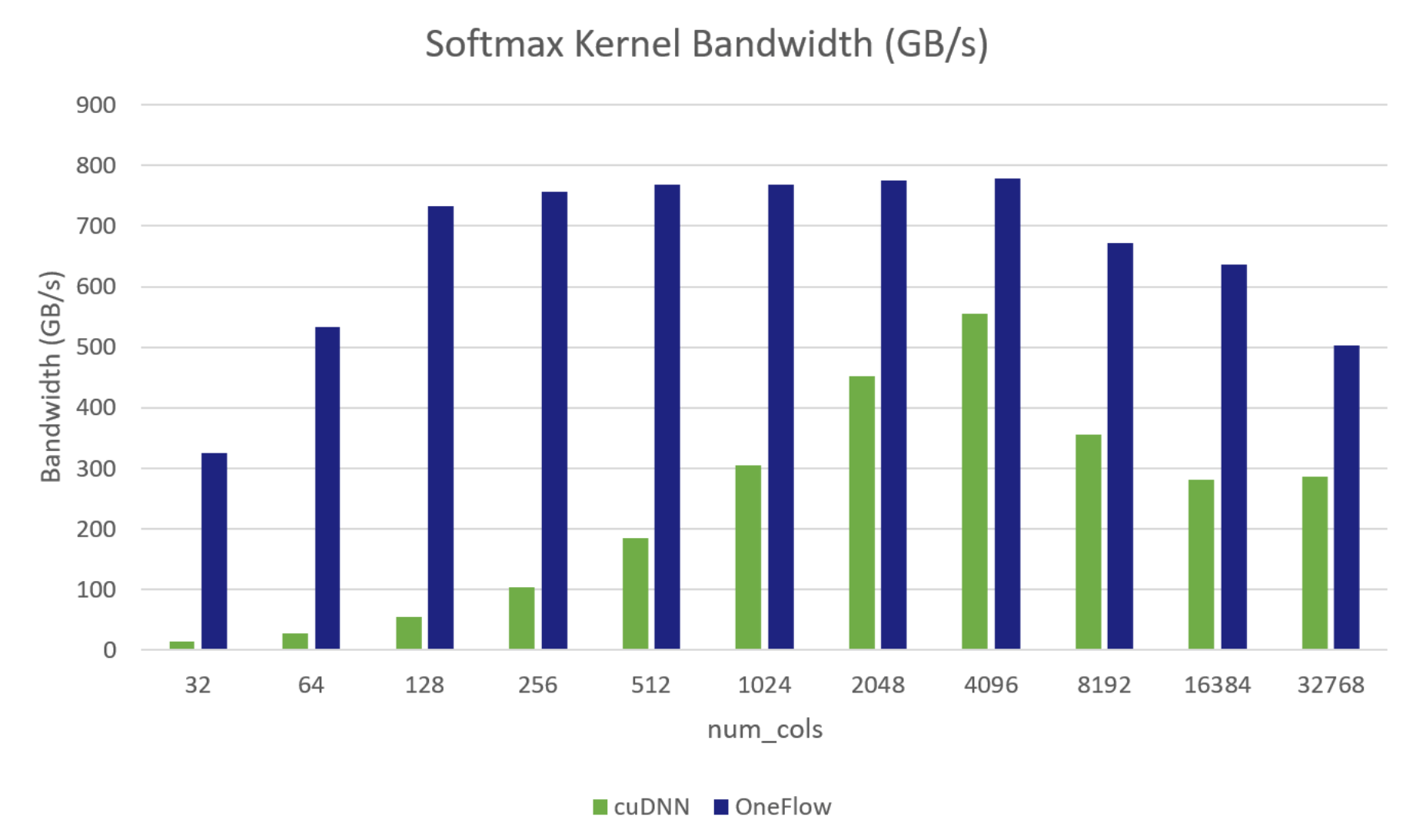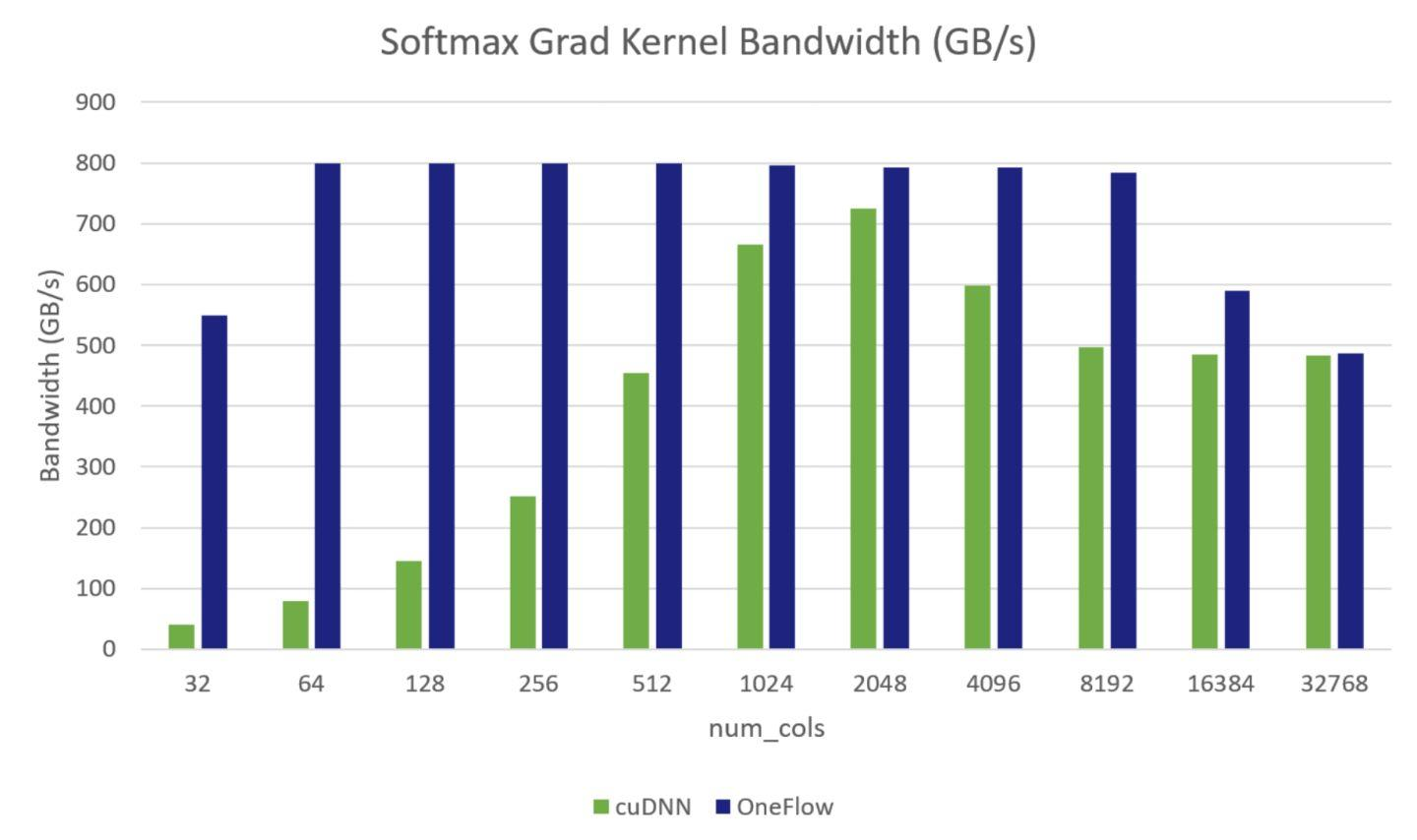# OneFlow深度優化Softmax CUDA Kernel的技巧

Softmax 函式的輸入形狀為:(num_rows, num_cols)，num_cols的變化，會對有效頻寬產生影響；因為，沒有一種 通用 的優化方法可以實現在所有 num_cols的情況下都是傳輸最優的。所以，在 OneFlow 中採用分段函式優化SoftmaxKernel：對於不同 num_cols範圍，選擇不同的實現，以在所有情況下都能達到較高的有效頻寬。見 Optimize softmax cuda kernel

OneFlow 中分三種實現，分段對 softmax 進行優化：

(1) 一個 Warp 處理一行的計算，適用於 num_cols <= 1024 情況

(2) 一個 Block 處理一行的計算，藉助 Shared Memory 儲存中間結果資料，適用於需要的 Shared Memory 資源滿足 Kernel Launch 的可啟動條件的情況，在本測試環境中是 1024 < num_cols <= 4096

(3) 一個 Block 處理一行的計算，不使用 Shared Memory，重複讀輸入 x，適用於不支援(1)、（2)的情況

# 實現1：每個Warp處理一行或兩行元素。

\

```template<template<typename> typename ReductionOp, typename T> __inline__ __device__ T WarpAllReduce(T val) { for (int mask = kWarpSize / 2; mask > 0; mask /= 2) { val = ReductionOp<T>()(val, __shfl_xor_sync(0xffffffff, val, mask)); } return val; }```

SoftmaxWarpImpl的實現有以下幾個模板引數：

LOAD、STORE分別代表輸入輸出，使用`load.template load<pack_size>(ptr, row_id, col_id);``store.template store<pack_size>(ptr, row_id, col_id);` 進行讀取和寫入。使用LOAD和STORE有兩個好處：1、可以在CUDA Kernel中只關心計算型別ComputeType，而不用關心具體的資料型別T。2、只需要加幾行程式碼就可以快速支援Softmax和其他Kernel Fuse，減少頻寬需求，提升整體效能。普通的SoftmaxKernel直接使用DirectLoad和DirectStore，FusedSoftmaxKernel如\ FusedScaleSoftmaxDropoutKernel只需要定義一個ScaleLoad結構和一個DropoutStore結構用於對輸入x做Scale預處理和對輸出y做Dropout後處理。

algorithm代表使用的演算法，可選項有Algorithm::kSoftmax或Algorithm::kLogSoftmax。

CUDA Kernel執行的主體迴圈邏輯如下，首先根據 num_cols資訊算出每個執行緒要處理的cols_per_thread，每個執行緒分配`rows_per_access * cols_per_thread`大小的暫存器，將輸入x讀到暫存器中，後續計算均從暫存器中讀取。

# pragma unroll

``````for (int row_id = 0; row_id < rows_per_access; ++row_id) {
ComputeType* row_buf = buf[row_id];
``````

# pragma unroll

``````  for (int pack_id = 0; pack_id < num_packs; ++pack_id) {
const int col = (pack_id * thread_group_width + lane_id) * pack_size;
if (!padding || col < cols) {
``````

# pragma unroll

``````      for (int i = 0; i < pack_size; ++i) {
}
} else {
``````

# pragma unroll

``````      for (int i = 0; i < pack_size; ++i) {
row_buf[pack_id * pack_size + i] = -Inf<ComputeType>();
}
}
}
}
ComputeType warp_max[rows_per_access];
``````

# pragma unroll

``````for (int row_id = 0; row_id < rows_per_access; ++row_id) {
}
``````

# pragma unroll

``````for (int row_id = 0; row_id < rows_per_access; ++row_id) {
ComputeType* row_buf = buf[row_id];
``````

# pragma unroll

``````  for (int i = 0; i < cols_per_thread; ++i) {
if (algorithm == Algorithm::kSoftmax) {
row_buf[i] = Exp(row_buf[i] - warp_max[row_id]);
} else if (algorithm == Algorithm::kLogSoftmax) {
row_buf[i] -= warp_max[row_id];
} else {
__trap();
}
}
}
ComputeType warp_sum[rows_per_access];
``````

# pragma unroll

``````for (int row_id = 0; row_id < rows_per_access; ++row_id) {
}
``````

# pragma unroll

``````for (int row_id = 0; row_id < rows_per_access; ++row_id) {
ComputeType* row_buf = buf[row_id];
``````

# pragma unroll

``````  for (int i = 0; i < cols_per_thread; ++i) {
if (algorithm == Algorithm::kSoftmax) {
row_buf[i] = Div(row_buf[i], warp_sum[row_id]);
} else if (algorithm == Algorithm::kLogSoftmax) {
row_buf[i] -= Log(warp_sum[row_id]);
} else {
__trap();
}
}
``````

# pragma unroll

``````  for (int i = 0; i < num_packs; ++i) {
const int col = (i * thread_group_width + lane_id) * pack_size;
if (!padding || col < cols) {
store.template store<pack_size>(row_buf + i * pack_size, row + row_id, col);
}
}
}
``````

} } ```

# 實現2： 一個Block處理一行元素

```template<template<typename> typename ReductionOp, typename T, int block_size> __inline__ __device__ T BlockAllReduce(T val) { typedef cub::BlockReduce<T, block_size> BlockReduce; __shared__ typename BlockReduce::TempStorage temp_storage; __shared__ T result_broadcast; T result = BlockReduce(temp_storage).Reduce(val, ReductionOp<T>()); if (threadIdx.x == 0) { result_broadcast = result; } __syncthreads(); return result_broadcast; }```

CUDA Kernel的各個模板引數在(1)中做了詳細介紹，執行的主體迴圈邏輯如下，根據 num_cols算出需要的 Shared Memory 大小作為 Launch Kernel 引數，藉助 Shared Memory 儲存輸入，後續計算直接從 Shared Memory 讀取。

``` template global void SoftmaxBlockSMemImpl(LOAD load, STORE store, const int64_t rows, const int64_t cols) { extern shared align(sizeof(double)) unsigned char shared_buf[]; auto* buf = reinterpret_cast(shared_buf); const int tid = threadIdx.x; assert(cols % pack_size == 0); const int num_packs = cols / pack_size; for (int64_t row = blockIdx.x; row < rows; row += gridDim.x) { ComputeType thread_max = -Inf(); for (int pack_id = tid; pack_id < num_packs; pack_id += block_size) { ComputeType pack[pack_size]; load.template load(pack, row, pack_id * pack_size);

# pragma unroll

``````  for (int i = 0; i < pack_size; ++i) {
buf[i * num_packs + pack_id] = pack[i];
}
}
const ComputeType row_max = BlockAllReduce<MaxOp, ComputeType, block_size>(thread_max);
for (int col = tid; col < cols; col += block_size) {
if (algorithm == Algorithm::kSoftmax) {
const ComputeType exp_x = Exp(buf[col] - row_max);
buf[col] = exp_x;
} else {
const ComputeType x = buf[col] - row_max;
buf[col] = x;
}
}
const ComputeType row_sum = BlockAllReduce<SumOp, ComputeType, block_size>(thread_sum);
for (int pack_id = tid; pack_id < num_packs; pack_id += block_size) {
ComputeType pack[pack_size];
``````

# pragma unroll

``````  for (int i = 0; i < pack_size; ++i) {
if (algorithm == Algorithm::kSoftmax) {
pack[i] = Div(buf[i * num_packs + pack_id], row_sum);
} else if (algorithm == Algorithm::kLogSoftmax) {
pack[i] = buf[i * num_packs + pack_id] - Log(row_sum);
} else {
__trap();
}
}
store.template store<pack_size>(pack, row, pack_id * pack_size);
}
``````

} } ```

# 實現3：一個Block處理一行的元素，不使用Shared Memory，重複讀輸入x

``` template global void SoftmaxBlockUncachedImpl(LOAD load, STORE store, const int64_t rows, const int64_t cols) { const int tid = threadIdx.x; assert(cols % pack_size == 0); const int num_packs = cols / pack_size; for (int64_t row = blockIdx.x; row < rows; row += gridDim.x) { ComputeType thread_max = -Inf(); for (int pack_id = tid; pack_id < num_packs; pack_id += block_size) { ComputeType pack[pack_size]; load.template load(pack, row, pack_id * pack_size);

# pragma unroll

``````  for (int i = 0; i < pack_size; ++i) { thread_max = max(thread_max, pack[i]); }
}
const ComputeType row_max = BlockAllReduce<MaxOp, ComputeType, block_size>(thread_max);
for (int pack_id = tid; pack_id < num_packs; pack_id += block_size) {
ComputeType pack[pack_size];
``````

# pragma unroll

``````  for (int i = 0; i < pack_size; ++i) { thread_sum += Exp(pack[i] - row_max); }
}
const ComputeType row_sum = BlockAllReduce<SumOp, ComputeType, block_size>(thread_sum);
for (int pack_id = tid; pack_id < num_packs; pack_id += block_size) {
ComputeType pack[pack_size];
``````

# pragma unroll

``````  for (int i = 0; i < pack_size; ++i) {
if (algorithm == Algorithm::kSoftmax) {
pack[i] = Div(Exp(pack[i] - row_max), row_sum);
} else if (algorithm == Algorithm::kLogSoftmax) {
pack[i] = (pack[i] - row_max) - Log(row_sum);
} else {
__trap();
}
}
store.template store<pack_size>(pack, row, pack_id * pack_size);
}
``````

} } ```

# 公共優化技巧

1、將 Half 型別 pack 成 Half2 進行存取，在不改變延遲情況下提高指令吞吐，類似 CUDA template for element-wise kernels 的優化

2、Shared Memory 中的 Bank Conflicts

CUDA 將 Shared Memory 按照4位元組或8位元組大小劃分到32個 bank 中，對於 Volta 架構是4位元組，這裡以4位元組為例，如下圖所示，0-128 bytes地址分別在bank 0-31中，128-256也分別在 bank0-31中。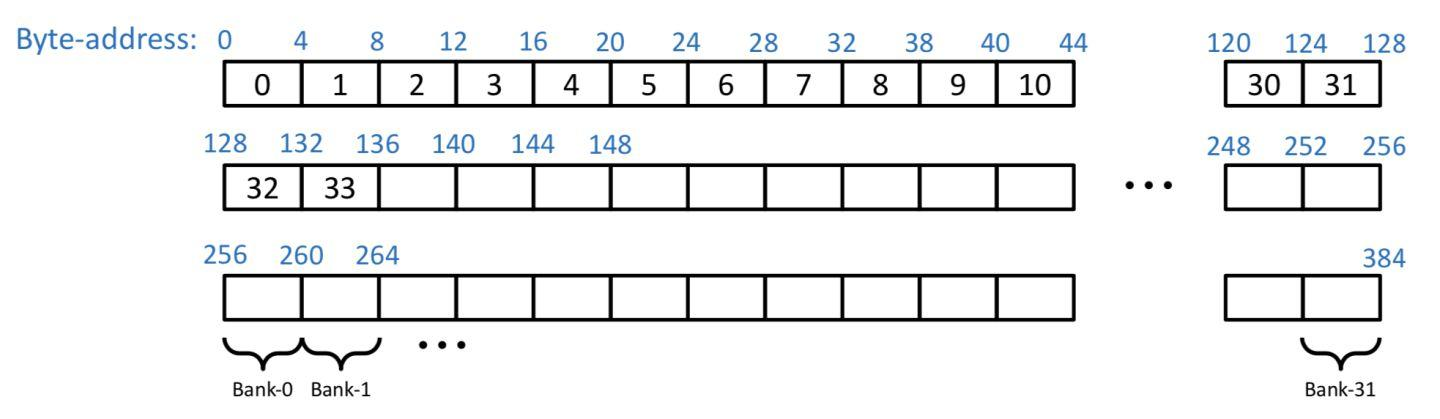：此圖及以下 Bank Conflicts 圖片來自VOLTA Architecture and performance optimization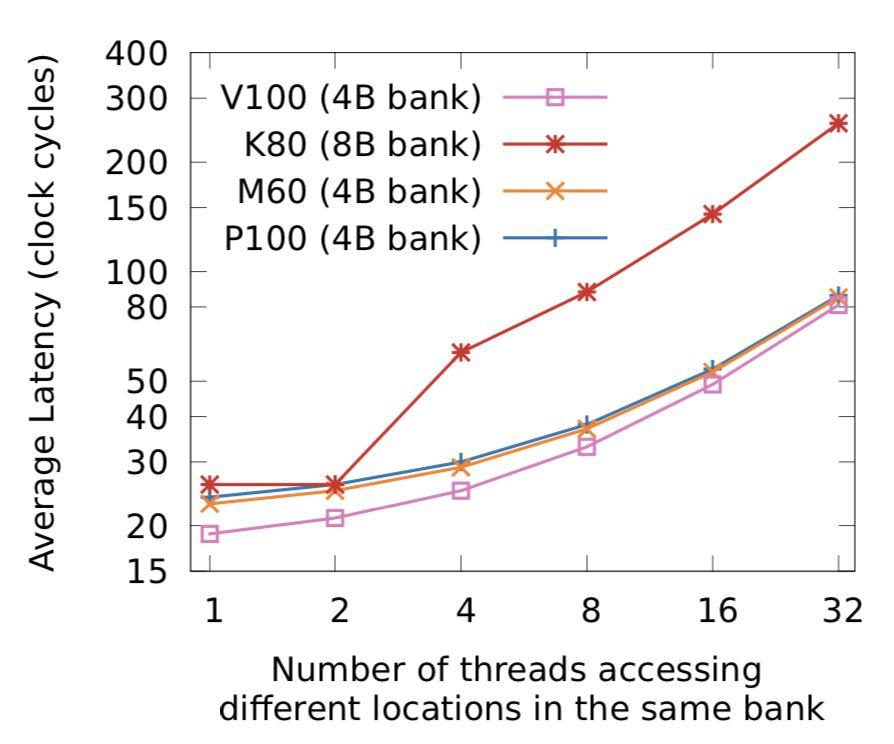：圖來自Dissecting the NVIDIA Volta GPU Architecture via Microbenchmarking

Bank Conflicts 的幾種情況：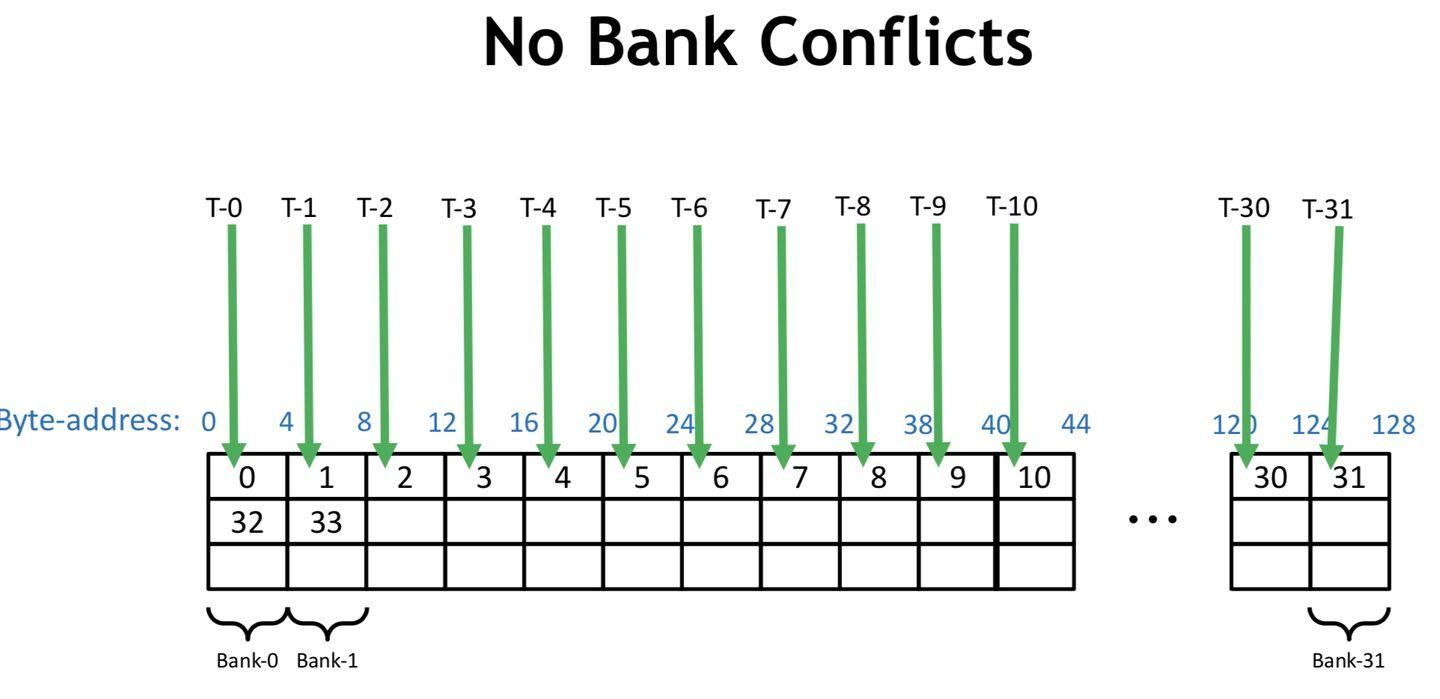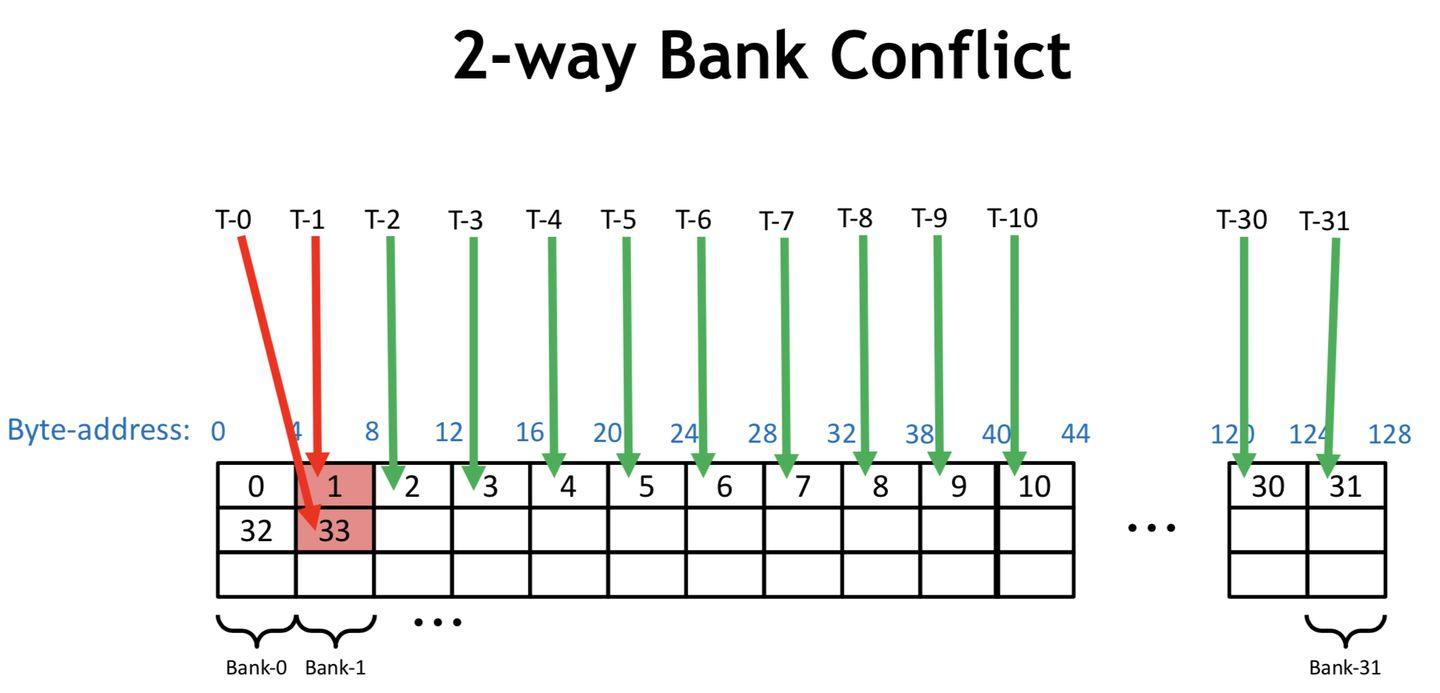```

# pragma unroll

``````  for (int j = 0; j < pack_size; ++j) {
buf[pack_id * pack_size * j] = pack[j];
}
``````

```

```

# pragma unroll

``````  for (int j = 0; j < pack_size; ++j) {
buf[num_packs * j + pack_id] = pack[j];
}
``````

```

# 參考資料：

Using CUDA Warp-Level Primitives | NVIDIA Developer Blog

CUDA Pro Tip: Increase Performance with Vectorized Memory Access

Dissecting the NVIDIA Volta GPU Architecture via Microbenchmarking

VOLTA Architecture and performance optimization